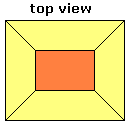Spike's Calculators

# Volume And Area Truncated Rectangle Pyramid

Calculate the volume and the surface area of a truncated rectangular pyramid.This calculator works for any measurement unit. For example, if you calculate inches, inches will have to be used in all the length fields, and the result of the areas will be square inches; the volume results in cubic inches and length measurements in the starting unit, inches.

### Truncated Rectangular Pyramid Volume

Upper Base Length #
Upper Base Width #
Lower Base Length #
Lower Base Width #
Height #
Decimal Precision #

#### Results:

 Volume #³ Base Area #² Lateral Area #² Surface Area #² Surface to Volume Ratio sa:vol

#### Calculation

• the length of the upper base (l)
• the width of the upper base (w)
• the length of the lower base (L)
• the width of the lower base (W)
• the height of the truncated pyramid
• decimal precision, number of digits after the decimal point

#### Results

• the truncated pyramid volume (unit cubed)
• the base area of the pyramid (bottom and top) (unit squared)
• the lateral area (unit squared)
• the surface area (unit squared)
• surface area to volume ratio (decimal)
##### Formula
```V = H/6(Lw+Wl+2(lw+LW))
B = LW+lw
LA = 2((L+l)/2e+(W+w)/2f))
e = (W-w)²+H²
f = (L-l)²+H²
SA = LA+BA
s/v = SA/V
where V is the volume of the truncated rectangle pyramid
B the base area (top and bottom)
LA the lateral area
SA the surface area
s/v the surface area to volume ratio
```